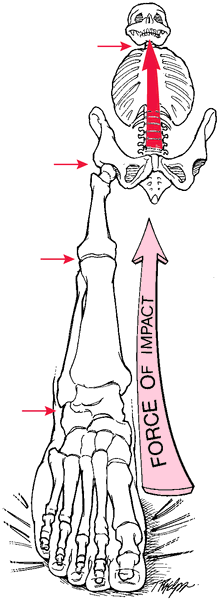# electromotive force

Also found in: Dictionary, Thesaurus, Legal, Acronyms, Encyclopedia, Wikipedia.
Related to electromotive force: electromotive force series

## force

[fors]
energy or power; that which originates or arrests motion or other activity.Forces resulting from a fall are transmitted up to the spine through the long leg bones and pelvis. From McQuillan et al., 2002.
electromotive force the force that, by reason of differences in potential, causes a flow of electricity from one place to another, giving rise to an electric current.
reserve force energy above that required for normal functioning. In the heart it is the power that will take care of the additional circulatory burden imposed by bodily exertion.
shearing f's see shear.
van der Waals f's the relatively weak, short-range forces of attraction existing between atoms and molecules, which results in the attraction of nonpolar organic compounds to each other (hydrophobic bonding).
Miller-Keane Encyclopedia and Dictionary of Medicine, Nursing, and Allied Health, Seventh Edition. © 2003 by Saunders, an imprint of Elsevier, Inc. All rights reserved.

## e·lec·tro·mo·tive force (EMF),

the force (measured in volts) that causes the flow of electricity from one point to another.
Farlex Partner Medical Dictionary © Farlex 2012

## e·lec·tro·mo·tive force

(EMF) (ĕ-lek'trō-mō'tiv fōrs)
The force (measured in volts) that causes the flow of electricity from one point to another.
Medical Dictionary for the Health Professions and Nursing © Farlex 2012

## e·lec·tro·mo·tive force

(EMF) (ĕ-lek'trō-mō'tiv fōrs)
The force (measured in volts) that causes electricity to flow.
Medical Dictionary for the Dental Professions © Farlex 2012
References in periodicals archive ?
Knowing the value of the teslametric constant, we deduced the effective value of the magnetic field from the measured effective value of the electromotive force.
Nevertheless, current variation of BLDC motors can also be obtained using counter electromotive force instead of Hall sensor effects.
In addition, he generalizes the definition of the electromotive intensity or electromotive force to the force on unit electric charges moving with velocity v.
Case 3 (when electromotive force is in terms of unit step function, i.e., E(t) = u(t)).
where V is the relation coefficient of the main parameters, D is the armature diameter, [l.sub.ef] is the effective length of armature, [a'.sub.p] is the pole-arc coefficient, [K.sub.Nm] is the waveform coefficient of air gap magnetic field, [K.sub.dp] is the winding factor, A is the line current density, [B.sub.[delta]] is the air gap flux density, [K.sub.E] is the ratio of induced electromotive force and terminal voltage in the rated load, [P.sub.N] is the rated power, cos [[phi].sub.N] is the power factor, and n is the rated speed of generator.
p Number of pole pair, f Supply frequency, Hz, p Magnetic permeability, H/m, [L.sub.[sigma]1f] Inductance corresponding to the part of the stator winding outside the stator magnetic core, H, [rho] Resistivity, [ohm]m, [J.sub.s] Current density in the stator slots, A/[m.sup.2], t Time, s, s Slip, between 0 and 1, [B.sub.x] Radial component of the magnetic flux density, Wb, [B.sub.y] Azimuth component of the magnetic flux density, Wb, emf Electromotive force, V, FFT Fast Fourier Transform, 0BC No broken rotor bar, 1BC Motor with one broken rotor bar, Ecc Motor with rotor eccentricity, REFERENCES
According to Random House Webster's College Dictionary, "tension" covers the act or state of stretching or straining, mental or emotional strain, intense, suppressed suspense, anxiety, or excitement, a strained relationship between individuals, groups, or nations, "the longitudinal deformation of an elastic body that results it its elongation, and electromotive force, potential (as in electricity).
This magnetic field induces electromotive force in the metal which in turn induces eddy currents.
where E, [E.sub.0], F, R, T, and a are electromotive force, standard electromotive force, Faraday constant (96 485.3399 C [mol.sup.-1]), gas constant (8.314 J/K mol), temperature, and activity, respectively.
The back electromotive force (EMF) is too small in the low speed, which is crucial to estimate.

Site: Follow: Share:
Open / Close